|

# 实景解析|富有人情味的现代美学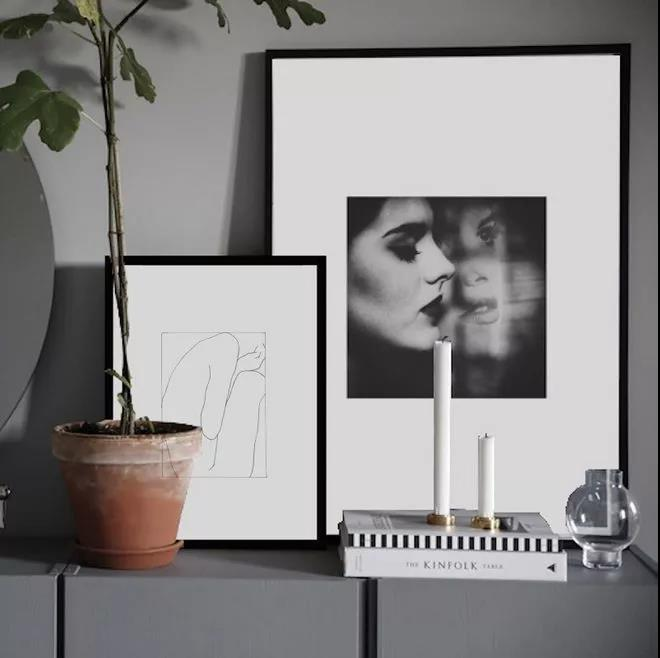【房 屋 类 型】洋 房

【项 目 面 积】180㎡

【设 计 风 格】现 代

【主 要 用 材】瑞士恩仕洁具、高德瓷砖、梦天整木等

中国文化博大精深，对它的爱是骨子里的东西，无法替代。有人喜欢它的意境，有人喜欢它的材质，也有人喜欢它的全部。

但是又希望要贴近如今简单又品质的生活。这就是设计之初主人对设计师的要求，看似具体，其实广泛。

一次次的沟通、一次次的纠结，一次次的自我调整，才出来今天的模样，

从当初决定选择星杰，到如今工程完美竣工，业主一直表示：选择星杰，就是选择了放心。

竣工时，一句“这就是我想象中家的样子”，一切值了。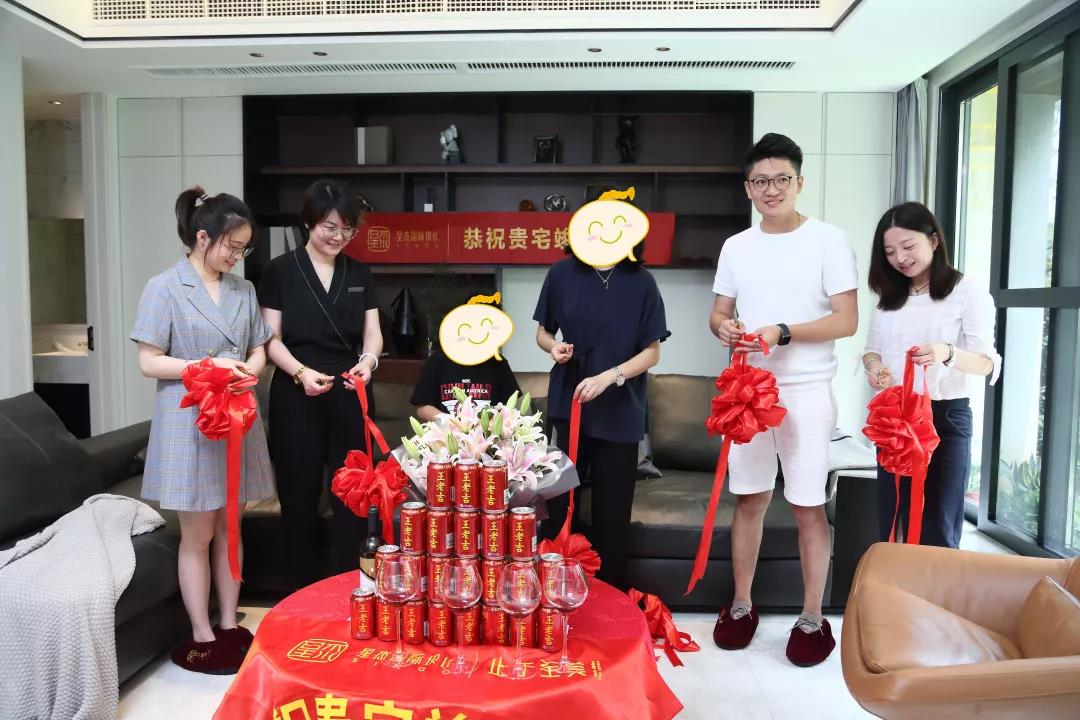品位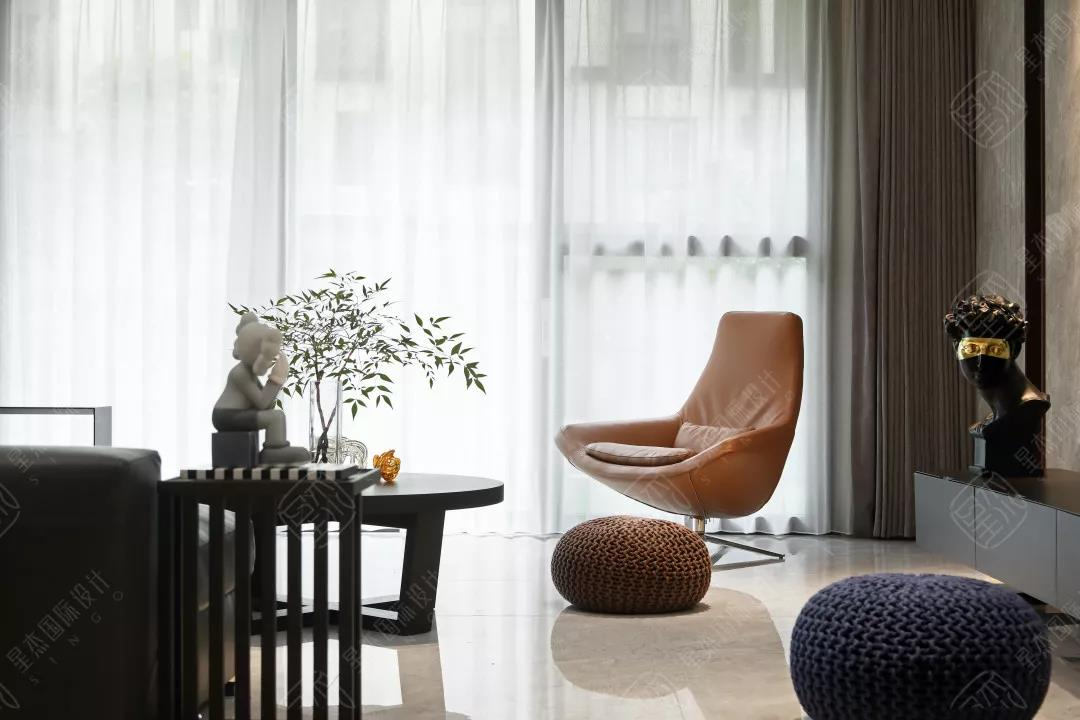现在越来越多的年轻业主选择摒弃繁复，拥抱更和世界接轨的现代风潮，追寻生活的轻盈质感。本案亦是如此，业主是一对年轻的80后私营业主，有两个可爱的儿子，崇尚简约主义，喜欢纯粹的现代空间，具有时尚的生活理念，他们对设计的创意度和时尚度有着很高的要求，这对设计师来说是一场不小的考验。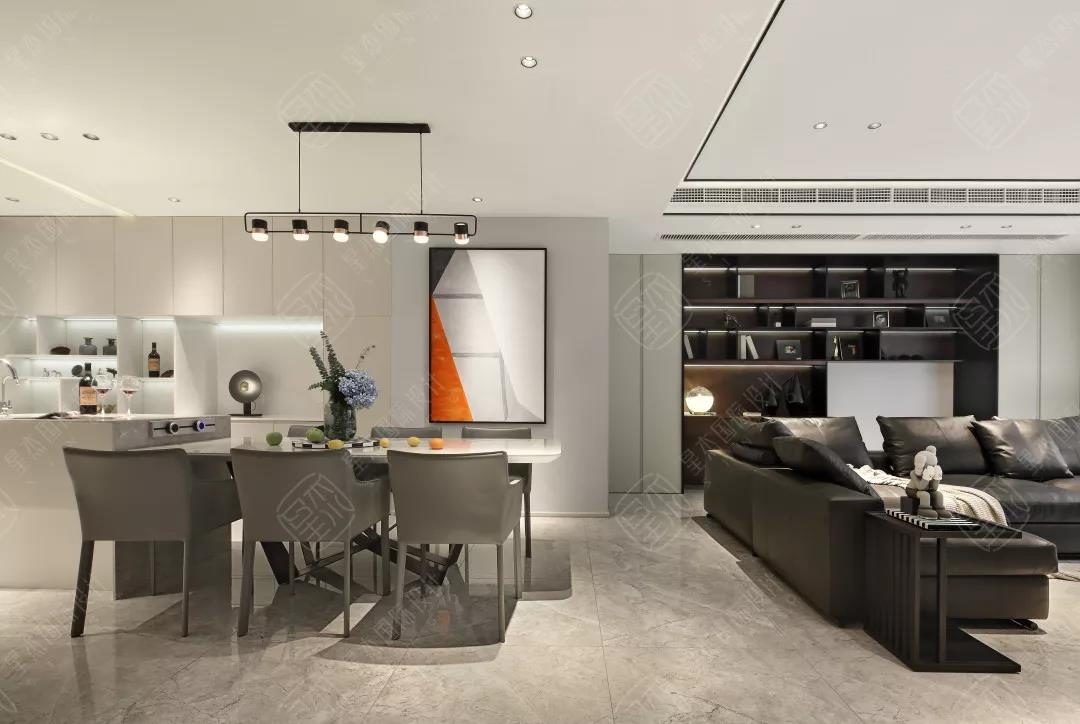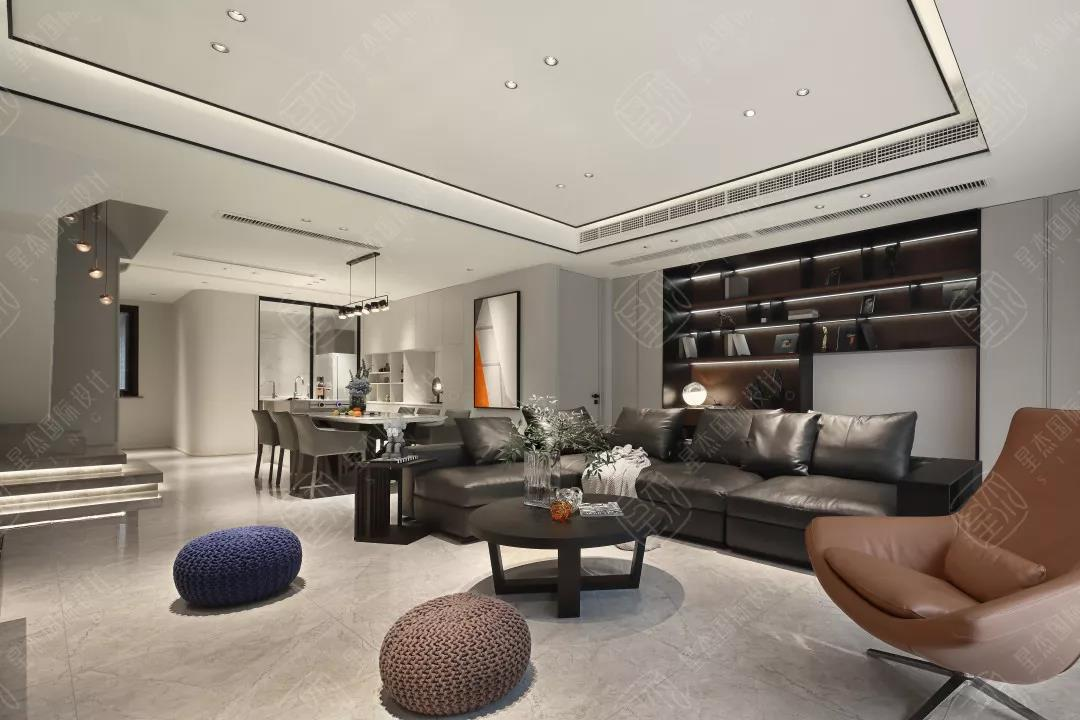所以，设计师在现代主义的设计思想之上，加入了“富有人情味”的现代美学，空间多采用「经典」设计，无论从硬装手法到软配装饰，都会有那种利落的简洁之美，然后用些许暖性的色彩点缀整个空间柔和的氛围，个性化的装饰体现了居住者和设计师的巧思，具有年轻人活力的生活属性。

把设计还给生活，可能是家装设计师需要深入研究的课题。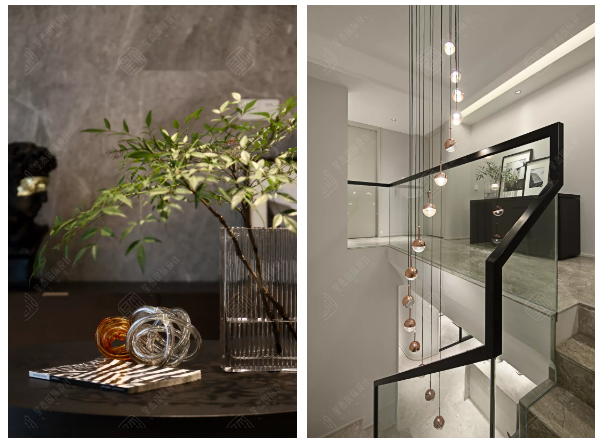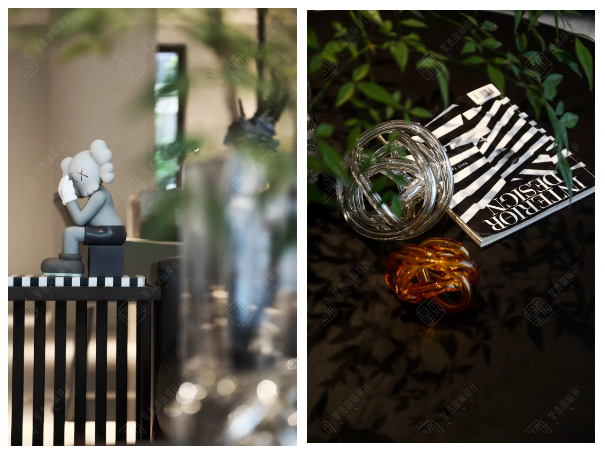业主在家追求休闲放松的感觉，在一层的动区空间都采用敞开式的表现形式，使业主能更加方便的照看小孩儿，客厅的大移门能很好的把花园的景色引入室内，与客厅融为一体。室内空间褪去繁重的墙面装饰，享受更加轻松自如的生活。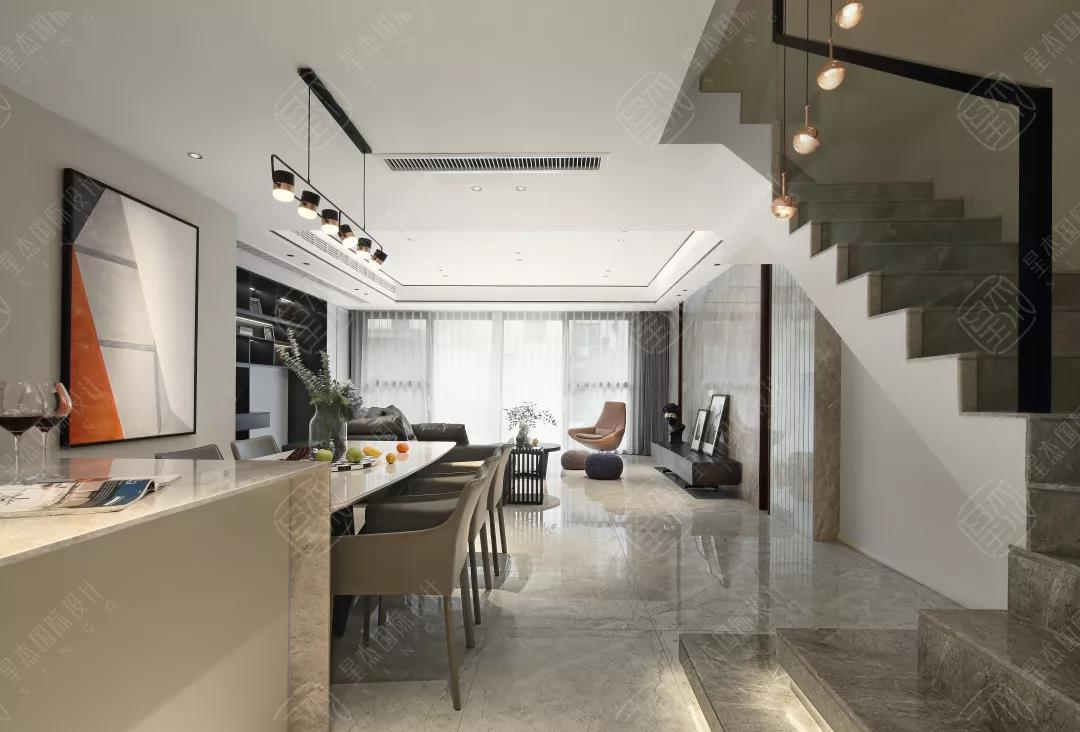憧 憬 的 日 子

『搬入新家大半年了，每天下班回到家仍是满心愉悦。常常一个人坐在客厅的单人椅上，望向花园景观到客厅，藤的黄、灯的白、高高低低的绿植，迎面是颇具创意的挂画，宁静平和……老公系着黑白条围裙在厨房忙碌，我隔着吧台为他送上一杯清茶……清晨时，儿子捧着书在阳台的吊篮里背诵着课文，晃晃悠悠、咿咿呀呀，身后是漫天的朝霞…… 』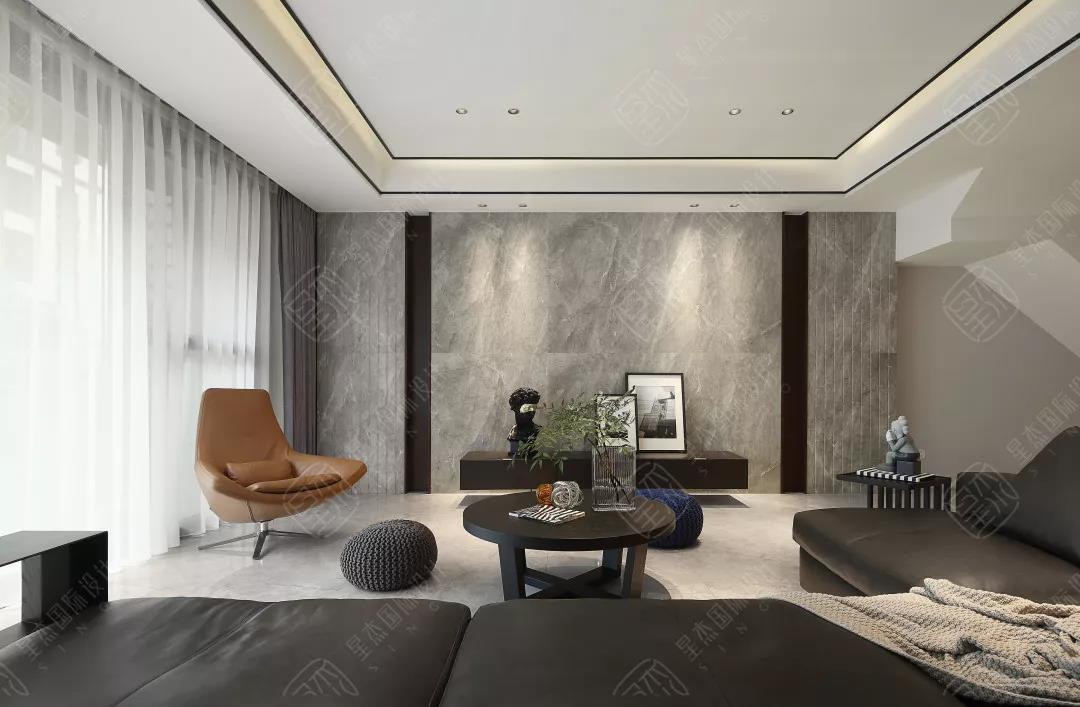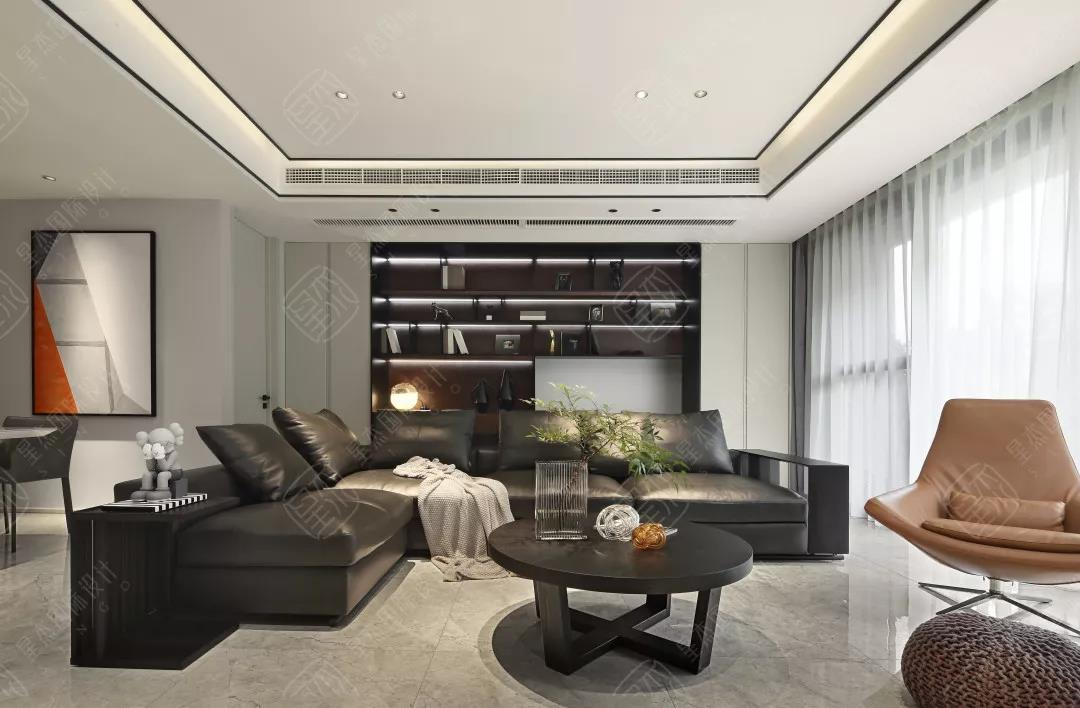在前期沟通时，设计师能感觉到业主对生活的热爱、对家庭的重视、以及对宝宝的疼爱。所以在空间点缀色上选择了温暖的橙色。橙色的组成元素中有鲜明的红色和灿烂的黄色，融合后产生的温馨与温暖正好是男女业主想要的有活力、有温度、有爱的生活状态。

客厅以极简灰为基调，简洁大气，明朗通透，与现代抽象艺术大卫雕塑摆件相衬，营造出一种怪诞的张力。即便是如此宽敞得可以尽情挥洒的空间，设计师依然谨慎克制，小心翼翼的衡量着每一个家具、每一个饰品的尺寸大小、摆放位置。在琐碎的生活经验基础之上，通过对元素、部品的细腻选择与创新，实践构建日常生活的同时，打造更具想象力的38°空间氛围。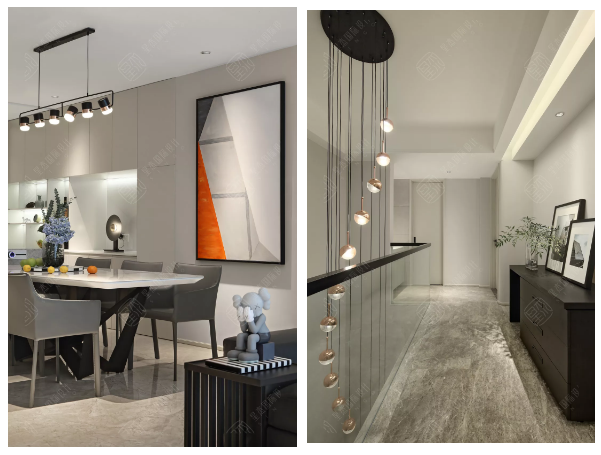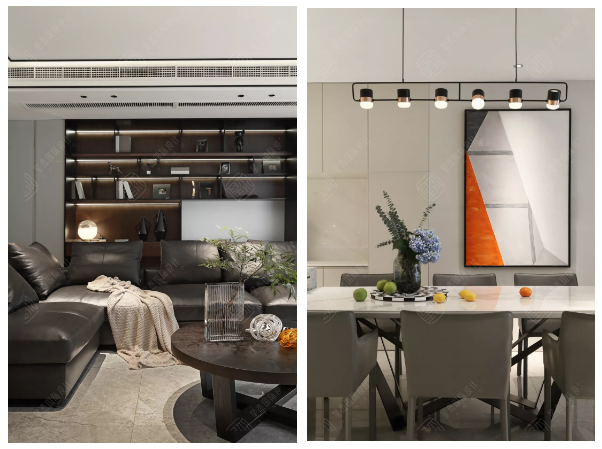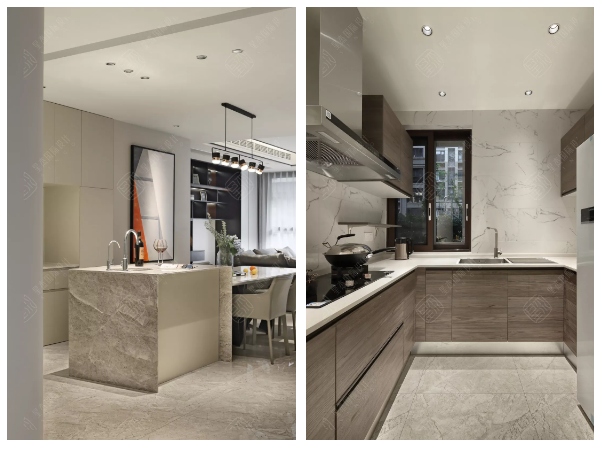开放式空间拥有开阔的视觉，让光线和空气相互流通蔓延。设计师打破固有格局，将厨房和餐厅统一于同一空间之下，模糊了区域间的界限，以多元和复合型的功能型来重新构建空间，弱化了以往对厨餐厅的理解，以一种国际化都市感的空间布局方式，引领一种全新的现代生活方式。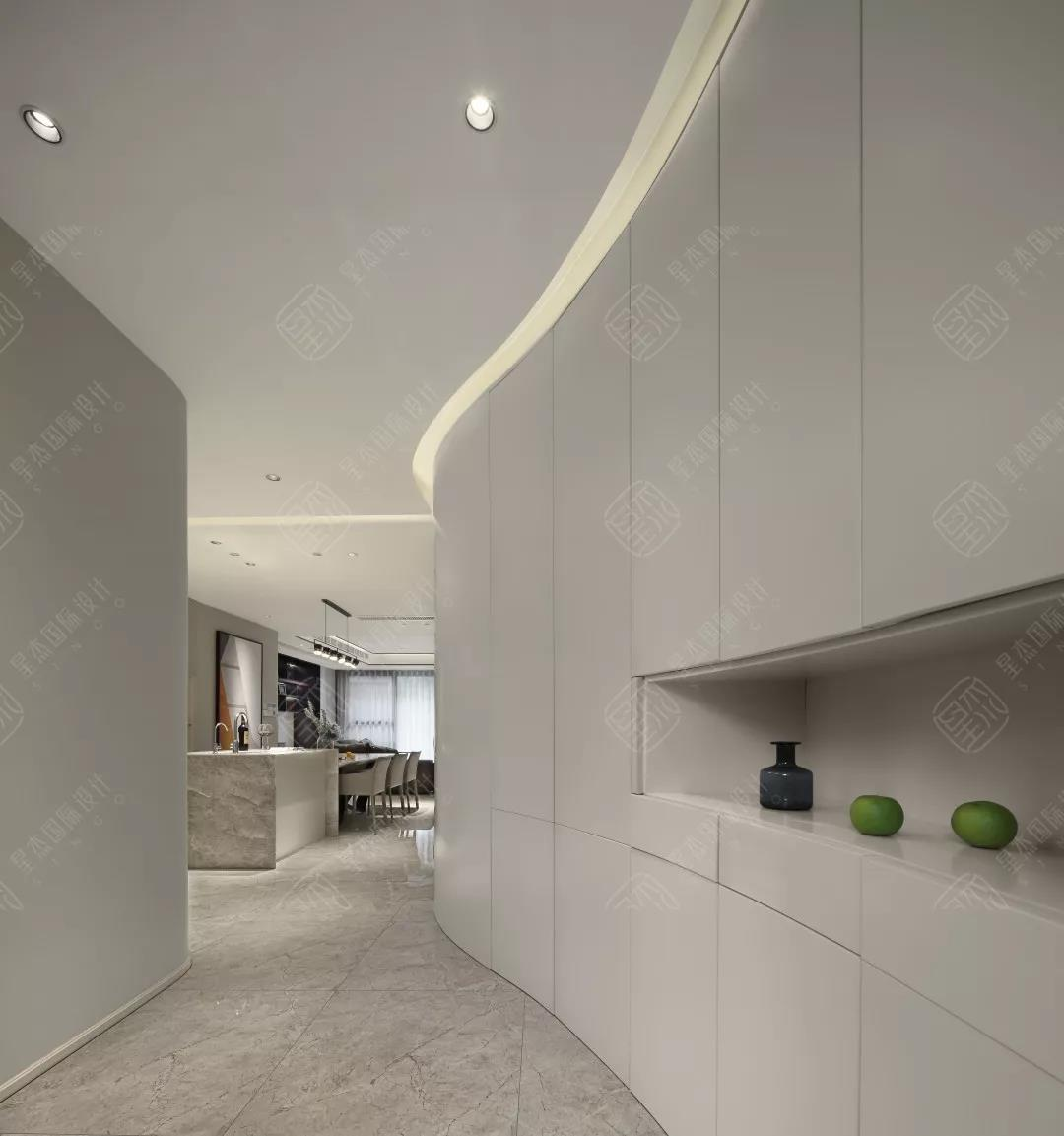装 修 前 后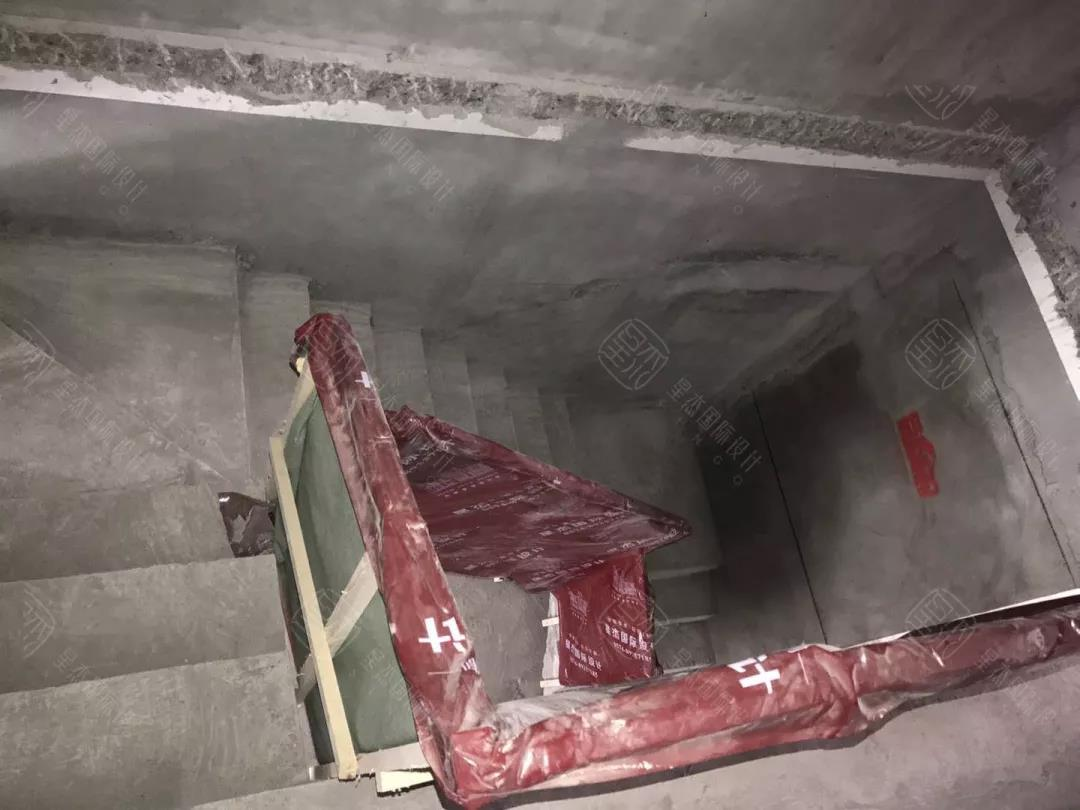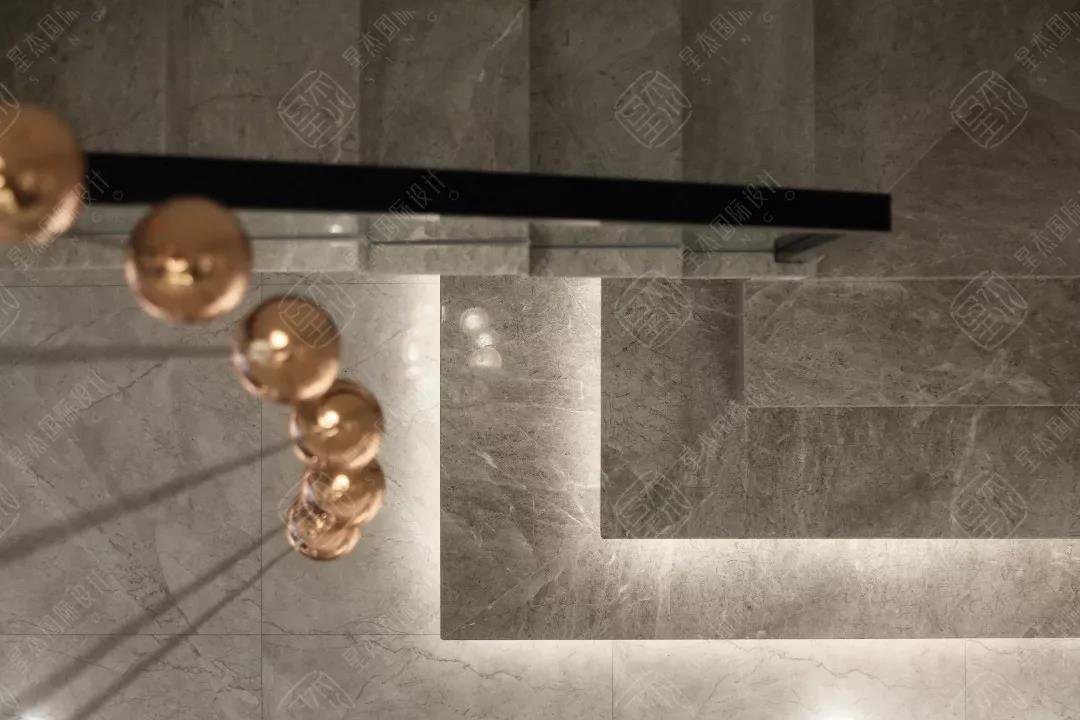原始图vs实景图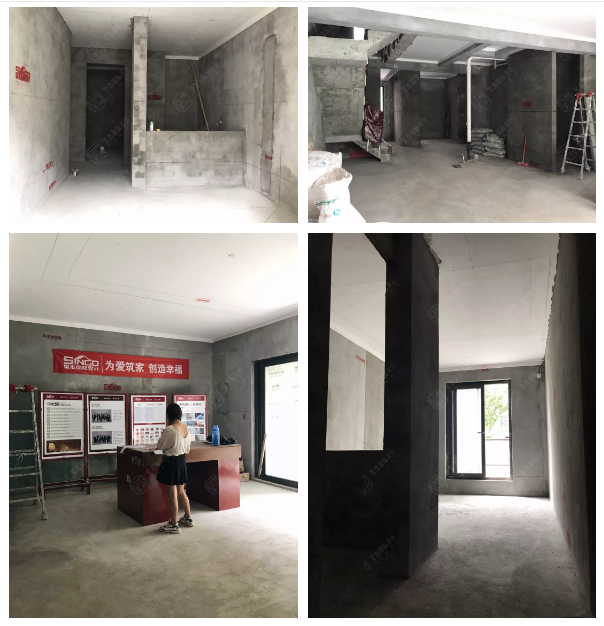▲装修时期 现场工地原始图

效果图vs实景图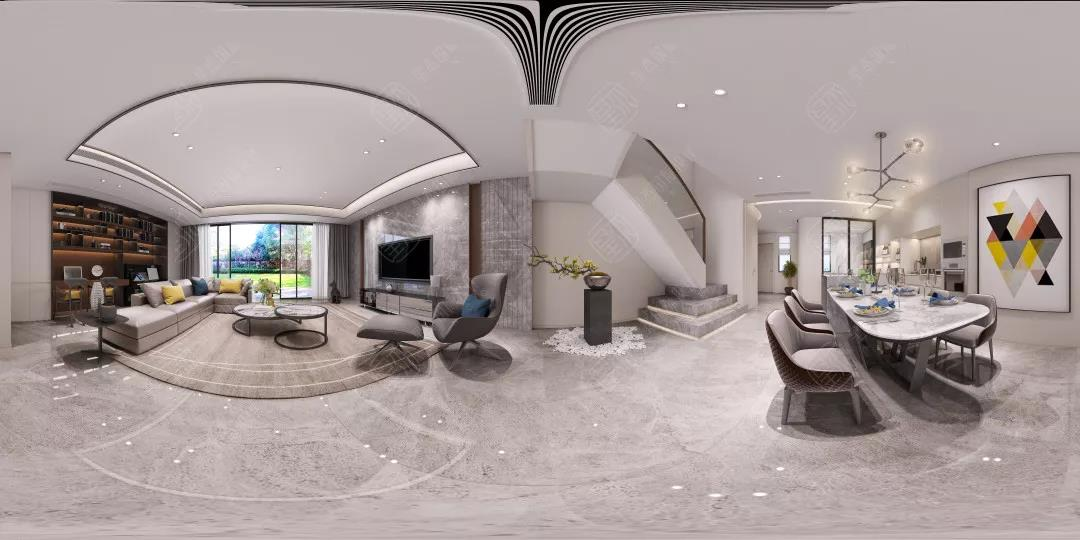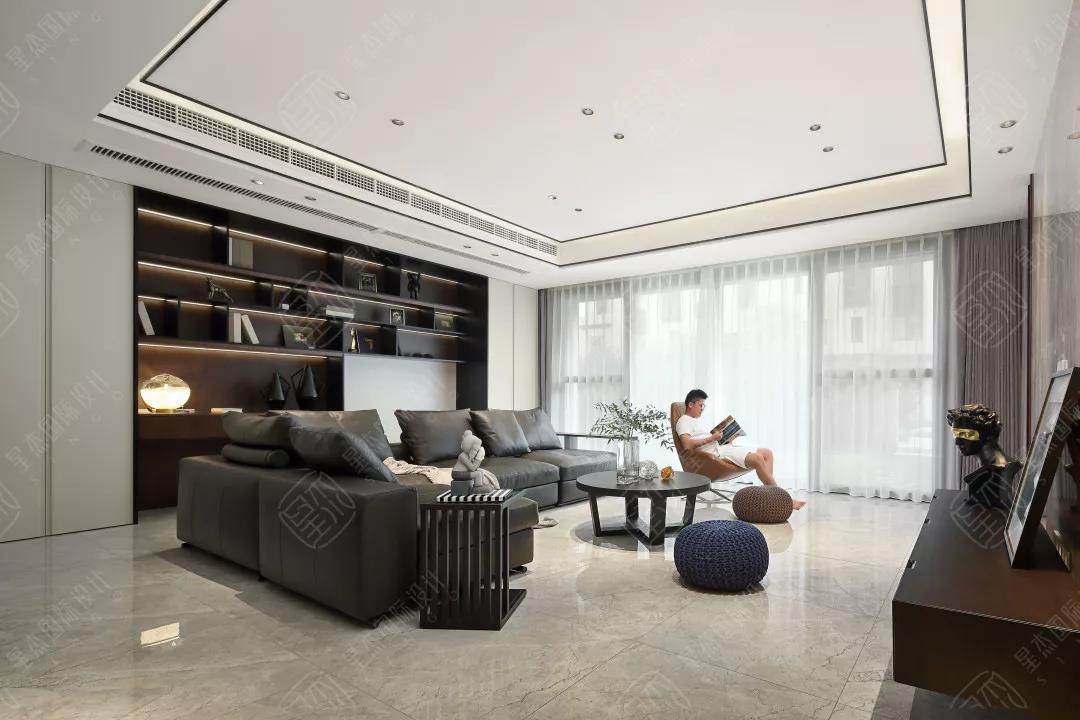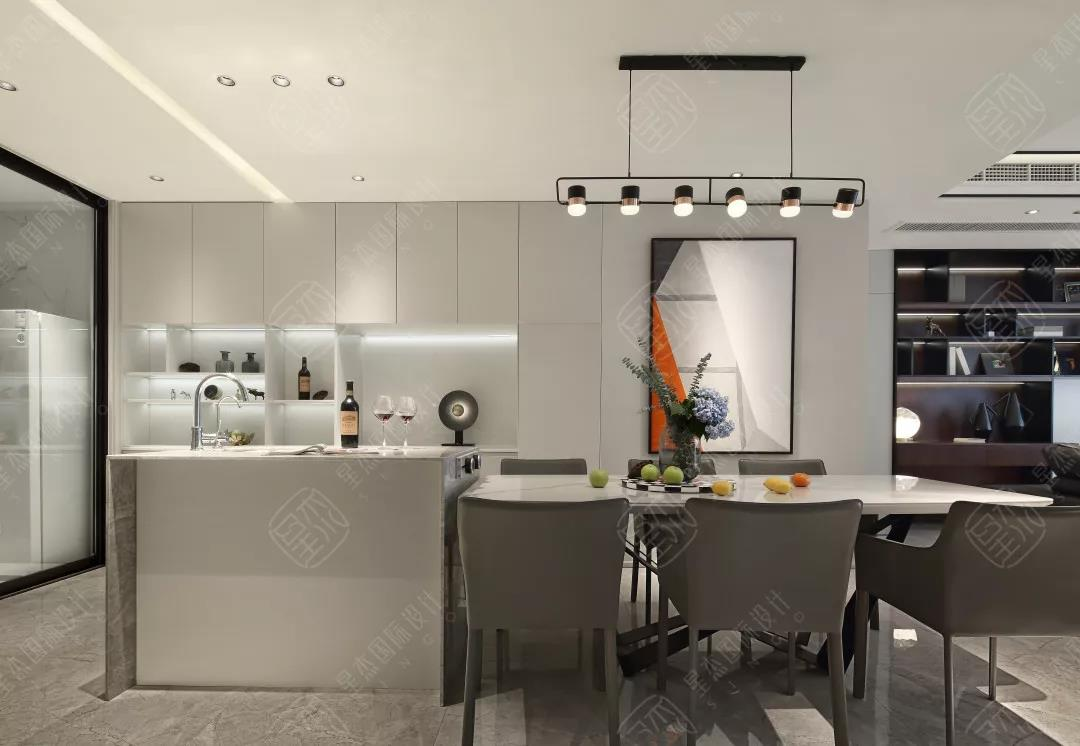▲客餐厅1:1高效还原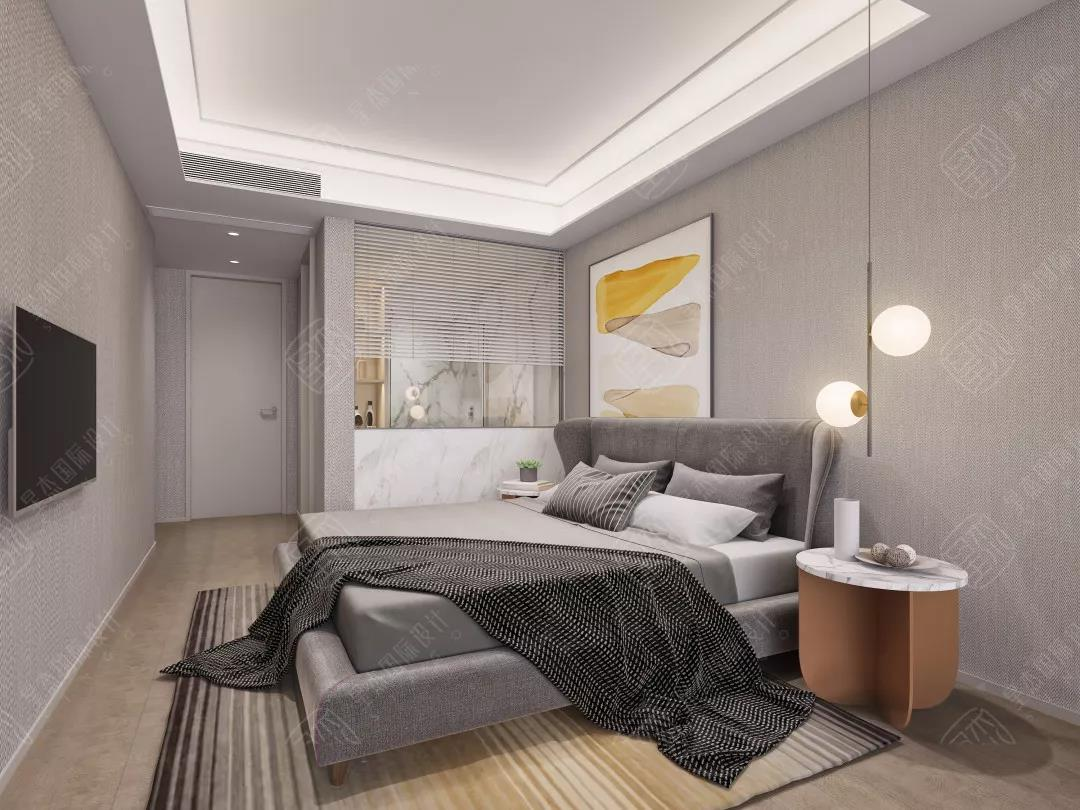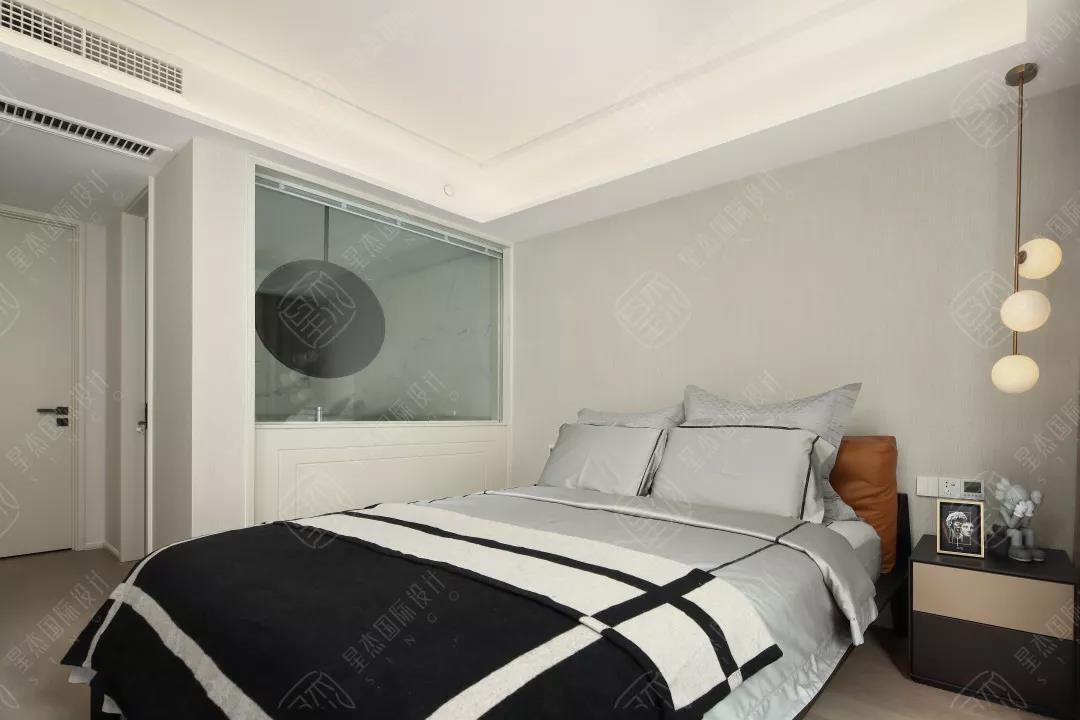▲主卧1:1高效还原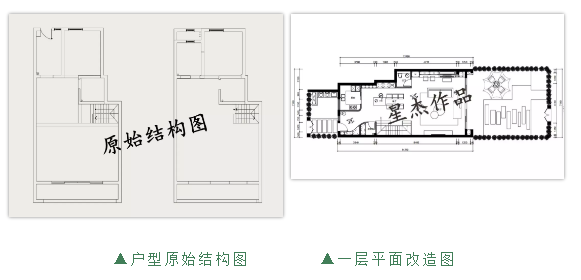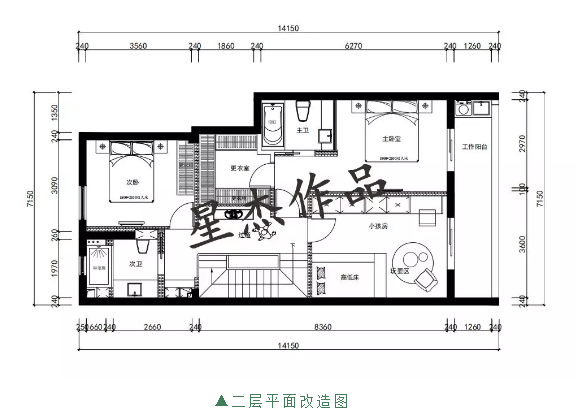正因为有星杰海派精工的保驾护航，使效果图到实景完美落地，品质、工期得到保障。“海派精工”项目管理系统，是真正以“客户服务”为核心理念贯穿项目全过程，无论是项目前期企划筹备、中期施工监控还是后期售后服务，既可掌控全程也可省心交托，确保工地，工期有保障，质量更无忧。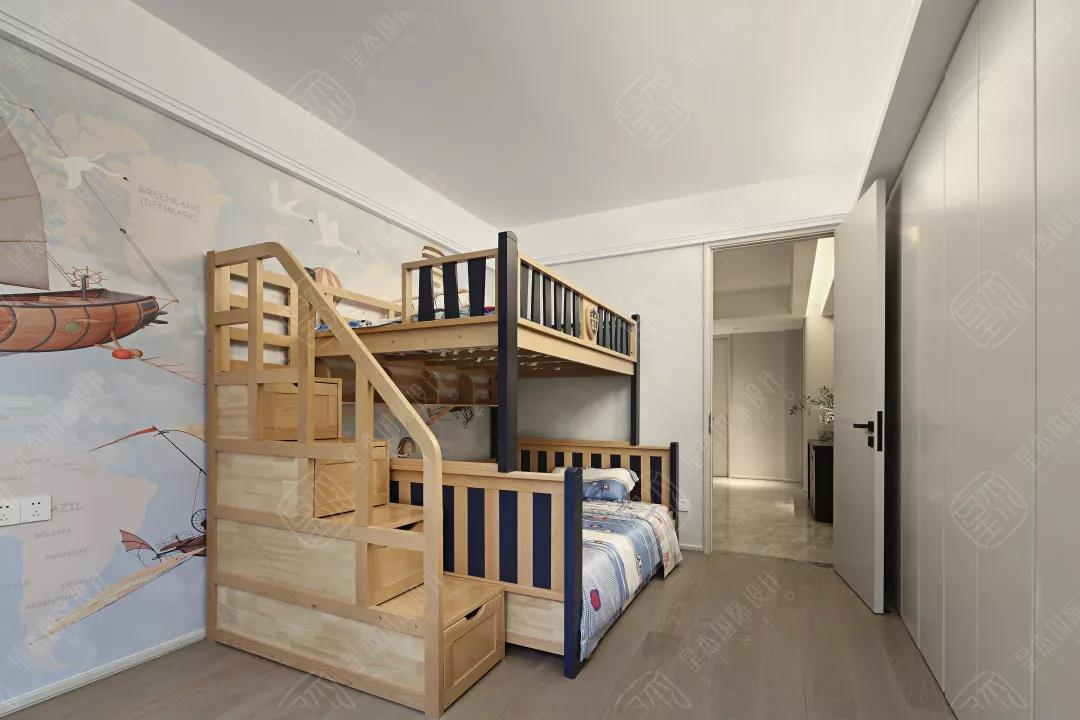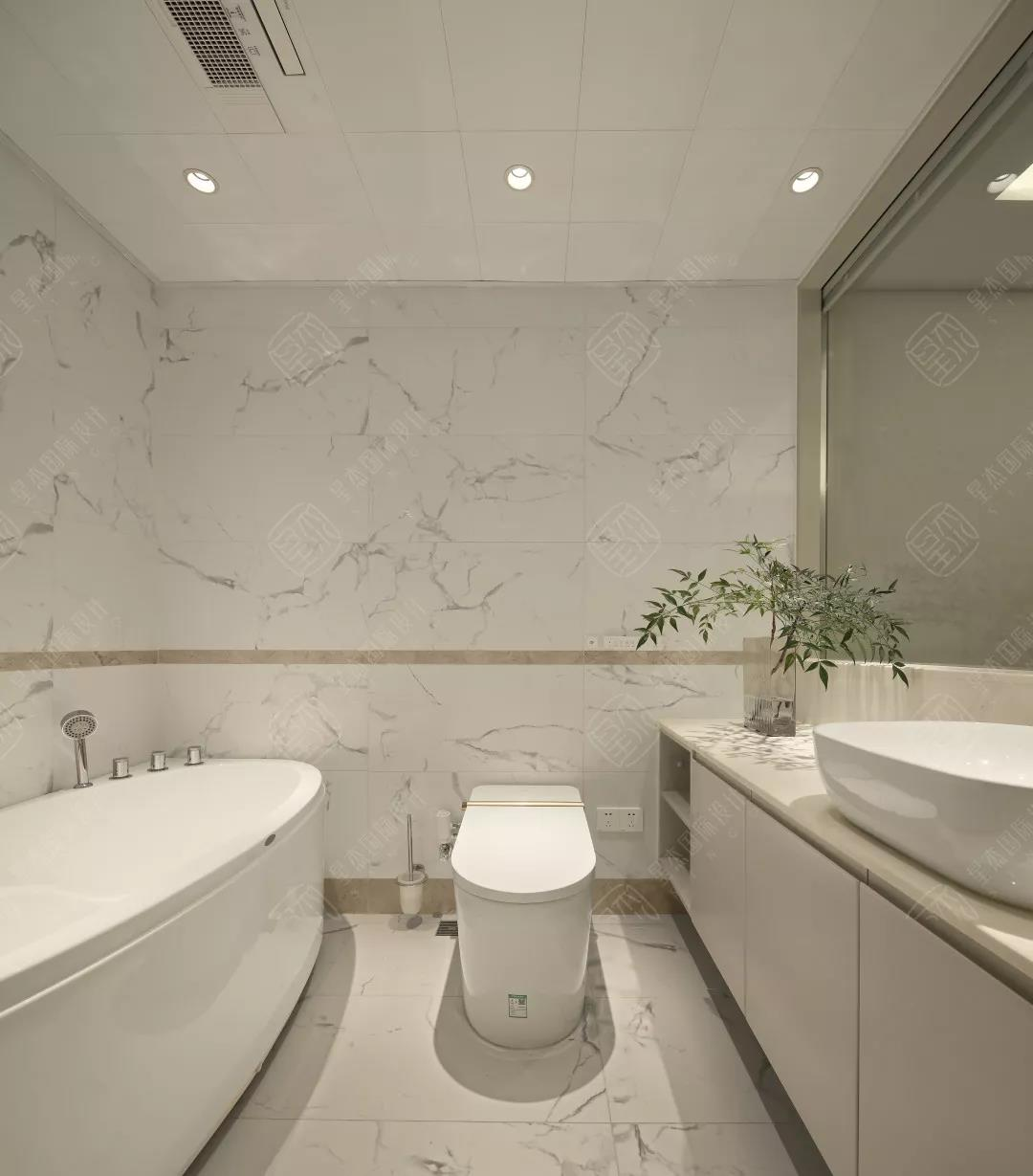整个项目过程中，业主对生活的乐此不疲是最感染人的。厨房的挂画、橱柜里的小摆件、过道的花瓶、沙发旁圆形造型的边几、电视柜的大卫雕塑、床边的小吊灯、甚至是展示柜里数不清的手办、儿子房墙面画及收纳梯……这些都是主人喜欢的东西、喜欢做的事。

有品质的简朴，有节制的丰盛，才是好设计的根本。

星杰

为高标配而生

量身定制最适合您的居住格局

星杰无锡六周年

星光璀璨，六载荣耀

四重豪礼回馈新客户

`声明：本文由入驻焦点开放平台的作者撰写，除焦点官方账号外，观点仅代表作者本人，不代表焦点立场错误信息举报电话： 400-099-0099，邮箱：jubao@vip.sohu.com，或点此进行意见反馈，或点此进行举报投诉。`A B C D E F G H J K L M N P Q R S T W X Y Z
A - B - C - D - E
• A
• 鞍山
• 安庆
• 安阳
• 安顺
• 安康
• 澳门
• B
• 北京
• 保定
• 包头
• 巴彦淖尔
• 本溪
• 蚌埠
• 亳州
• 滨州
• 北海
• 百色
• 巴中
• 毕节
• 保山
• 宝鸡
• 白银
• 巴州
• C
• 承德
• 沧州
• 长治
• 赤峰
• 朝阳
• 长春
• 常州
• 滁州
• 池州
• 长沙
• 常德
• 郴州
• 潮州
• 崇左
• 重庆
• 成都
• 楚雄
• 昌都
• 慈溪
• 常熟
• D
• 大同
• 大连
• 丹东
• 大庆
• 东营
• 德州
• 东莞
• 德阳
• 达州
• 大理
• 德宏
• 定西
• 儋州
• 东平
• E
• 鄂尔多斯
• 鄂州
• 恩施
F - G - H - I - J
• F
• 抚顺
• 阜新
• 阜阳
• 福州
• 抚州
• 佛山
• 防城港
• G
• 赣州
• 广州
• 桂林
• 贵港
• 广元
• 广安
• 贵阳
• 固原
• H
• 邯郸
• 衡水
• 呼和浩特
• 呼伦贝尔
• 葫芦岛
• 哈尔滨
• 黑河
• 淮安
• 杭州
• 湖州
• 合肥
• 淮南
• 淮北
• 黄山
• 菏泽
• 鹤壁
• 黄石
• 黄冈
• 衡阳
• 怀化
• 惠州
• 河源
• 贺州
• 河池
• 海口
• 红河
• 汉中
• 海东
• I
• J
• 晋中
• 锦州
• 吉林
• 鸡西
• 佳木斯
• 嘉兴
• 金华
• 景德镇
• 九江
• 吉安
• 济南
• 济宁
• 焦作
• 荆门
• 荆州
• 江门
• 揭阳
• 金昌
• 酒泉
• 嘉峪关
K - L - M - N - P
• K
• 开封
• 昆明
• 昆山
• L
• 廊坊
• 临汾
• 辽阳
• 连云港
• 丽水
• 六安
• 龙岩
• 莱芜
• 临沂
• 聊城
• 洛阳
• 漯河
• 娄底
• 柳州
• 来宾
• 泸州
• 乐山
• 六盘水
• 丽江
• 临沧
• 拉萨
• 林芝
• 兰州
• 陇南
• M
• 牡丹江
• 马鞍山
• 茂名
• 梅州
• 绵阳
• 眉山
• N
• 南京
• 南通
• 宁波
• 南平
• 宁德
• 南昌
• 南阳
• 南宁
• 内江
• 南充
• P
• 盘锦
• 莆田
• 平顶山
• 濮阳
• 攀枝花
• 普洱
• 平凉
Q - R - S - T - W
• Q
• 秦皇岛
• 齐齐哈尔
• 衢州
• 泉州
• 青岛
• 清远
• 钦州
• 黔南
• 曲靖
• 庆阳
• R
• 日照
• 日喀则
• S
• 石家庄
• 沈阳
• 双鸭山
• 绥化
• 上海
• 苏州
• 宿迁
• 绍兴
• 宿州
• 三明
• 上饶
• 三门峡
• 商丘
• 十堰
• 随州
• 邵阳
• 韶关
• 深圳
• 汕头
• 汕尾
• 三亚
• 三沙
• 遂宁
• 山南
• 商洛
• 石嘴山
• T
• 天津
• 唐山
• 太原
• 通辽
• 铁岭
• 泰州
• 台州
• 铜陵
• 泰安
• 铜仁
• 铜川
• 天水
• 天门
• W
• 乌海
• 乌兰察布
• 无锡
• 温州
• 芜湖
• 潍坊
• 威海
• 武汉
• 梧州
• 渭南
• 武威
• 吴忠
• 乌鲁木齐
X - Y - Z
• X
• 邢台
• 徐州
• 宣城
• 厦门
• 新乡
• 许昌
• 信阳
• 襄阳
• 孝感
• 咸宁
• 湘潭
• 湘西
• 西双版纳
• 西安
• 咸阳
• 西宁
• 仙桃
• 西昌
• Y
• 运城
• 营口
• 盐城
• 扬州
• 鹰潭
• 宜春
• 烟台
• 宜昌
• 岳阳
• 益阳
• 永州
• 阳江
• 云浮
• 玉林
• 宜宾
• 雅安
• 玉溪
• 延安
• 榆林
• 银川
• Z
• 张家口
• 镇江
• 舟山
• 漳州
• 淄博
• 枣庄
• 郑州
• 周口
• 驻马店
• 株洲
• 张家界
• 珠海
• 湛江
• 肇庆
• 中山
• 自贡
• 资阳
• 遵义
• 昭通
• 张掖
• 中卫

1室1厅1厨1卫1阳台

1
2
3
4
5

0
1
2

1

1

0
1
2
3报名成功，资料已提交审核A B C D E F G H J K L M N P Q R S T W X Y Z
A - B - C - D - E
• A
• 鞍山
• 安庆
• 安阳
• 安顺
• 安康
• 澳门
• B
• 北京
• 保定
• 包头
• 巴彦淖尔
• 本溪
• 蚌埠
• 亳州
• 滨州
• 北海
• 百色
• 巴中
• 毕节
• 保山
• 宝鸡
• 白银
• 巴州
• C
• 承德
• 沧州
• 长治
• 赤峰
• 朝阳
• 长春
• 常州
• 滁州
• 池州
• 长沙
• 常德
• 郴州
• 潮州
• 崇左
• 重庆
• 成都
• 楚雄
• 昌都
• 慈溪
• 常熟
• D
• 大同
• 大连
• 丹东
• 大庆
• 东营
• 德州
• 东莞
• 德阳
• 达州
• 大理
• 德宏
• 定西
• 儋州
• 东平
• E
• 鄂尔多斯
• 鄂州
• 恩施
F - G - H - I - J
• F
• 抚顺
• 阜新
• 阜阳
• 福州
• 抚州
• 佛山
• 防城港
• G
• 赣州
• 广州
• 桂林
• 贵港
• 广元
• 广安
• 贵阳
• 固原
• H
• 邯郸
• 衡水
• 呼和浩特
• 呼伦贝尔
• 葫芦岛
• 哈尔滨
• 黑河
• 淮安
• 杭州
• 湖州
• 合肥
• 淮南
• 淮北
• 黄山
• 菏泽
• 鹤壁
• 黄石
• 黄冈
• 衡阳
• 怀化
• 惠州
• 河源
• 贺州
• 河池
• 海口
• 红河
• 汉中
• 海东
• I
• J
• 晋中
• 锦州
• 吉林
• 鸡西
• 佳木斯
• 嘉兴
• 金华
• 景德镇
• 九江
• 吉安
• 济南
• 济宁
• 焦作
• 荆门
• 荆州
• 江门
• 揭阳
• 金昌
• 酒泉
• 嘉峪关
K - L - M - N - P
• K
• 开封
• 昆明
• 昆山
• L
• 廊坊
• 临汾
• 辽阳
• 连云港
• 丽水
• 六安
• 龙岩
• 莱芜
• 临沂
• 聊城
• 洛阳
• 漯河
• 娄底
• 柳州
• 来宾
• 泸州
• 乐山
• 六盘水
• 丽江
• 临沧
• 拉萨
• 林芝
• 兰州
• 陇南
• M
• 牡丹江
• 马鞍山
• 茂名
• 梅州
• 绵阳
• 眉山
• N
• 南京
• 南通
• 宁波
• 南平
• 宁德
• 南昌
• 南阳
• 南宁
• 内江
• 南充
• P
• 盘锦
• 莆田
• 平顶山
• 濮阳
• 攀枝花
• 普洱
• 平凉
Q - R - S - T - W
• Q
• 秦皇岛
• 齐齐哈尔
• 衢州
• 泉州
• 青岛
• 清远
• 钦州
• 黔南
• 曲靖
• 庆阳
• R
• 日照
• 日喀则
• S
• 石家庄
• 沈阳
• 双鸭山
• 绥化
• 上海
• 苏州
• 宿迁
• 绍兴
• 宿州
• 三明
• 上饶
• 三门峡
• 商丘
• 十堰
• 随州
• 邵阳
• 韶关
• 深圳
• 汕头
• 汕尾
• 三亚
• 三沙
• 遂宁
• 山南
• 商洛
• 石嘴山
• T
• 天津
• 唐山
• 太原
• 通辽
• 铁岭
• 泰州
• 台州
• 铜陵
• 泰安
• 铜仁
• 铜川
• 天水
• 天门
• W
• 乌海
• 乌兰察布
• 无锡
• 温州
• 芜湖
• 潍坊
• 威海
• 武汉
• 梧州
• 渭南
• 武威
• 吴忠
• 乌鲁木齐
X - Y - Z
• X
• 邢台
• 徐州
• 宣城
• 厦门
• 新乡
• 许昌
• 信阳
• 襄阳
• 孝感
• 咸宁
• 湘潭
• 湘西
• 西双版纳
• 西安
• 咸阳
• 西宁
• 仙桃
• 西昌
• Y
• 运城
• 营口
• 盐城
• 扬州
• 鹰潭
• 宜春
• 烟台
• 宜昌
• 岳阳
• 益阳
• 永州
• 阳江
• 云浮
• 玉林
• 宜宾
• 雅安
• 玉溪
• 延安
• 榆林
• 银川
• Z
• 张家口
• 镇江
• 舟山
• 漳州
• 淄博
• 枣庄
• 郑州
• 周口
• 驻马店
• 株洲
• 张家界
• 珠海
• 湛江
• 肇庆
• 中山
• 自贡
• 资阳
• 遵义
• 昭通
• 张掖
• 中卫• 手机• 分享
• 设计
免费设计
• 计算器
装修计算器
• 入驻
合作入驻
• 联系
联系我们
• 置顶
返回顶部